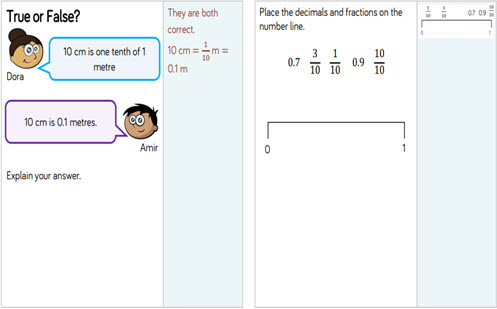# Fractions: tenths, equivalence and applying operations

This guide provides a sequence of small steps for deepening understanding of fractions, from using models to partition a whole into fractional parts through to finding the fraction of an amount, and adding and subtracting fractions.

Year level(s) Year 3, Year 4, Year 5
Audience Teacher
Purpose Teaching resource, Teaching strategies
Teaching strategies and pedagogical approaches Questioning, Concrete Representational Abstract model, Explicit teaching
Keywords equivalent fractions, comparing, ordering, adding fractions, subtracting fractions, bar model, explicit teaching

## Curriculum alignment

Curriculum connections Numeracy
Strand and focus Number, Build understanding
AC: Mathematics (V9.0) content descriptions
AC9M3N02

Recognise and represent unit fractions including 12, 13, 14, 15 and 110 and their multiples in different ways; combine fractions with the same denominator to complete the whole

AC9M4N04
Count by fractions including mixed numerals; locate and represent these fractions as numbers on number lines

AC9M4N01
Recognise and extend the application of place value to tenths and hundredths and use the conventions of decimal notation to name and represent decimals

AC9M5N03
Compare and order fractions with the same and related denominators including mixed numerals, applying knowledge of factors and multiples; represent these fractions on a number line

AC9M5N05
Solve problems involving addition and subtraction of fractions with the same or related denominators, using different strategies

Numeracy progression Interpreting fractions (P5, P6, P8)
Counting processes (P8)
Number and place value (P7)